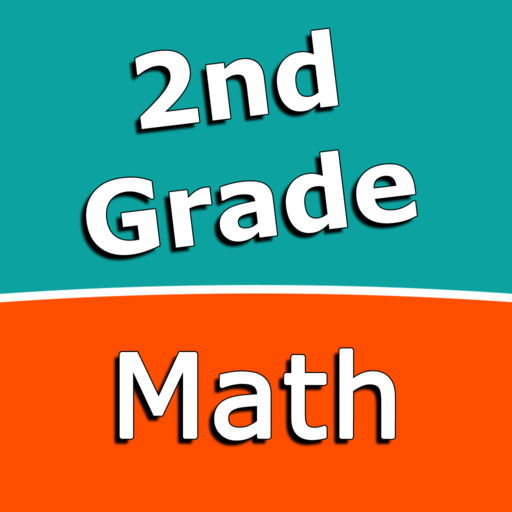Second Grade Math Trainer# Second Grade Math Trainer

## Sergey Malugin

1.0 for iPhone, iPad
\$1.99
0

0 Ratings

Release Date

2017-11-29

Size

28.6 MB

### Screenshots for iPhone

iPhone
More Screenshots for iPad
Second Grade Math Trainer Description
Contains Addition, Subtraction and Multiplication & Division learning blocks.

With Second grade Math Addition you can practice and improve the following skills:
- Addition up to 20
- Addition up to 100
- Add three one-digit numbers
- Add a two-digit and a one-digit number
- Add two multiples of ten
- Add multiples of 10 or 100
- Add two two-digit numbers
- Add three numbers up to two digits eac
- Addition with three-digit numbers

With Second grade Math Subtraction you can practice and improve the following skills:
- Subtraction facts - numbers up to 20
- Subtract a one-digit number from a two-digit number
- Subtract two two-digit numbers
- Subtract multiples of 10
- Subtract multiples of 100
- Subtract multiples of 10 or 100
- Subtract three-digit numbers
- Balance subtraction equations

With Second Grade Math Multiplication & Division you can practice and improve the following skills:
- Multiplication tables for 2, 3 and 4
- Multiplication tables up to 5×5
- Multiplication tables for 2, 3, 4, 5, and 10
- Multiplication tables for 6, 7, 8, and 9
- Multiplication tables up to 10×10
- Divisors and quotients up to 5
- Divisors and quotients up to 10

Choose between two different modes:
- Math Trainer
- Math Shot mini-game

Math Trainer mode helps you to master the math facts, you can solve math problems at you own pace without any time constrain.

Math Shot is a math mini-game with fun and engaging game-play and as we all know learning through play and fun is more effective. The game difficulty adapts to player's skills and makes the game suitable for all ages. Math Shot helps students to develop math fact fluency - the result of consistent learning, repetition, and practice.
Second Grade Math Trainer 1.0 Update
2017-11-29 Version History
More
Price:
\$1.99
Version:
1.0
Size:
28.6 MB
Genre:
Education
Release Date:
2017-11-29
Developer:
Sergey Malugin
Language:
English
More
You May Also Like
Developer Apps